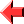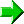﻿ TLWNN Tutorial Lesson 4: Arithmetic and Stuff#Lesson 4## The Library

Like most programming languages, TLWNN has its own runtime library.  TLWNN's library contains various procedures, many of which do things that cannot otherwise be done in TLWNN.  These procedures have – you've guessed it – no names!

The library is held on three packed stacks.  When a TLWNN program starts, the main stack is initialised to contain these stacks.

When using TLWNN's library, care should be taken to write code that will still work as the library expands.  Future TLWNN versions, should they ever happen, are likely both to add more procedures to the packed stacks and to add more packed stacks to the library.  But because any new stacks will be added at the bottom, and any new procedures will be added at the tops of the packed stacks (which, don't forget, become the bottoms when unpacked), it shouldn't matter too much as long as you're careful.

A full reference of the library procedures can be found in the specification.  The following sections explain some of the most commonly used library procedures and how to use them.

## Number Crunching

The topmost of the library stacks contains procedures that perform arithmetical operations.  (Well, one of them is logical rather than arithmetical, but we'll get to that later.)  Let's start with the bottom-most element of this stack, which performs subtraction.

One of the operands must be at the top of the main stack, and the other at the top of the auxiliary stack.  Of course, we need the subtraction procedure at the top of the main stack as well, but once that's been invoked, then the first operand will be at the top of the main stack ready for it.

For example, the following code

``````!105<62>!
``````

will subtract 62 from 105.  It works as follows:

1. The top-most of the library stacks is unpacked onto the auxiliary stack.  Remember that the procedure to subtract two numbers is now at the top of the auxiliary stack.
2. The number 105 is pushed to the main stack.
3. The library procedure for subtraction is moved from the auxiliary stack to the main stack.
4. The number 62 is pushed to the main stack, and then moved onto the auxiliary stack.
5. Subtraction is invoked.  The library procedure itself is popped, and then 105 and 62 are popped from the main and auxiliary stacks respectively.  The result is pushed to the main stack.

However, after this has executed, the library procedure that has been used is now lost for the rest of the execution of the program.  This is another place where being able to duplicate things is useful.

The second instruction from the bottom of the stack of arithmetic operations is division.  It works in the same way as subtraction - dividend on the main stack, divisor on the auxiliary stack.

With the aid of these, it is straightforward to implement addition and multiplication.

Next up is a procedure to divide one number by another and give the integer quotient and remainder.  The quotient is pushed to the main stack, and the remainder to the auxiliary stack.  The quotient is always rounded down, and the remainder always has the same sign as the divisor.

## Placing Conditions

The final element of the aforementioned stack of arithmetical procedures is used to do conditional stuff.  It relies on there being at least two objects in each stack, and the top-most of each being a number.

Put simply, it implements an if-then-else on a less-than comparison.  The values that are compared are the top-most stack elements, and the "then" and "else" parts are the elements just below these.  If the value popped off the main stack is less than the value popped off the auxiliary stack, choose what's next on the main stack, otherwise choose what's next on the auxiliary stack.

The reason it's not all that simple is that the chosen course of action is left on the same stack, while the one that isn't chosen is popped off.  (Of course, the numbers that were compared are popped as well.)  So you have to implement your own means of invoking it off the right stack.  One possible approach, if the idea is to invoke a procedure depending on the result of the comparison, is to have a procedure just below the selectable one on the main stack, which will invoke the procedure on the auxiliary stack if necessary.

This program:

``````Example eight
A simple conditional
81 105
0>>>!<<<<\*<<*>>\>\>\<<<!!
``````

will, for arbitrary values of 81 and 105, output the character corresponding to the smaller of the two numbers.  I encourage you to have a go at working out for yourself how it works.  (Hint: This code uses two instructions I haven't told you about yet.  `@` pops an object from the main stack and discards it, and `\` swaps the topmost elements of the main and auxiliary stacks.)

You'll notice that, in this case, the final state of the stacks depends on which option was chosen.  When programming real-world applications, you will often need to code up the actions so that this dependency is eliminated.

And then once you've got your head around that, have a go at implementing something resembling a for loop.  (The solution will appear later in the tutorial.)